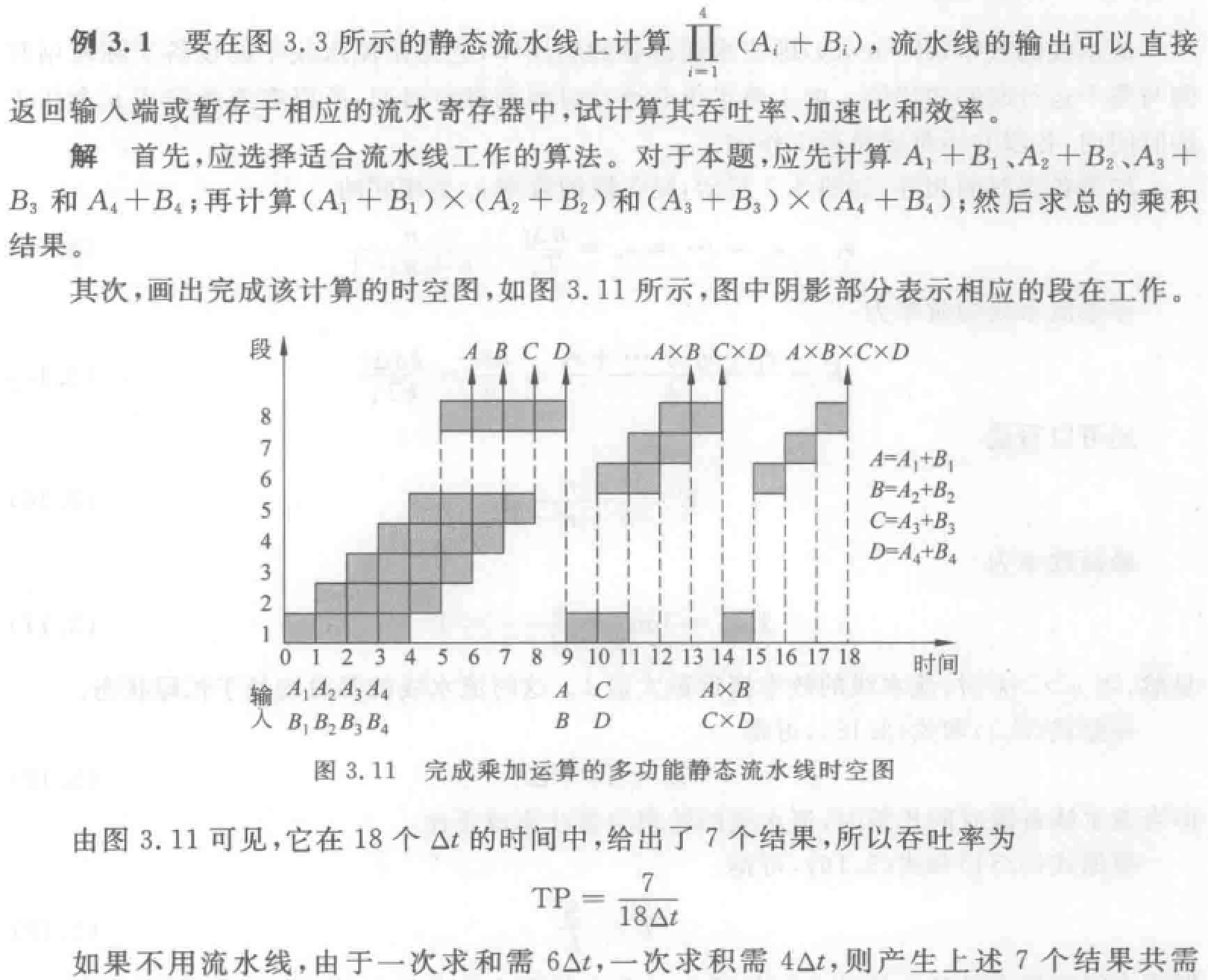# 系统结构笔记一、三章

## 第一章 基础知识

### 1.1基本概念(1)确定指令系统中是否有乘法指令属于计算机系统结构的内容,但乘法指令是用专门的乘法器实现,还是用加法器经多步操作来实现,属于计算机组成。而乘法器、加法器的物理实现﹐如器件的选定及所用的微组装技术等,属于计算机实现。

(2）主存容量与编址方式(按位、按字节或按字访问等)的确定属于计算机系统结构。为了达到给定的性能价格比,主存速度应多快、逻辑结构是否采用多体交叉等属于计算机组成。而主存系统的物理实现,如器件的选定、逻辑电路的设计,微组装技术的使用等均属于计算机实现。

• Flynn分类法：按照指令流和数据流的多倍性进行分类• 冯式分类法：用系统的最大并行度对计算机进行分类• Handler分类法：把计算机的硬件结构分成三个层次，并分别考虑它们的可并行-流水处理程度，将计算机用3对整数表示。
三个层次：### 1.2 计算机系统的设计

#### 1.2.1 计算机系统设计的定量原理

##### 大概率事件优先原理

（又叫经常性事件原则）: 优先加速使用频率高的部件。

• 为获得明显的系统性能。对大概率 (经常性)事件，
赋予优先处理权和资源使用权。
• 加快处理频繁出现事件对系统的影响远比加速
处理很少出现事件的影响。
##### Amdahl定律1. 部件加速比 $S_e$
2. 可改进比例 $F_e$
##### 程序的局部性原理
• 程序的时间局部性
• 程序即将用到的信息很可能就是目前正在使用的信息。
• 程序的空间局部
• 程序即将用到的信息很可能与目前正在使用的信息在空间上相邻或者临近。
##### CPU性能公式

$$CPU_{时间} = IC \times CPI \times t$$
$$= \sum (CPI_i \times IC_i)$$

$IC$:执行程序的指令条数
$CPI$:指令的平均时钟周期数

#### 1.2.2 计算机系统设计的主要内容

1. 需求分析
2. 软硬件功能划分： 经常使用的部分使用硬件加速
3. 前瞻性

### 1.2.3 系统设计的主要方法

1. top-down 设计
2. bottom-up 设计
3. middle-out 设计： 一般认为这个啥best的

### 1.3 计算机系统的性能评测

#### 指标

1. 执行时间
2. 速度
• MIPS 每秒百万条指令数 million instructions per second
• MFLOPS 每秒百万次浮点运算次数 million floating point operations per second
##### MIPS

$MIPS = I_{N} /(T_E \times 10^6)$
$=I_{N} /(I_{N} \times CPI \times t \times 10^6)$
$=1 / (CPI \times 10^6)$

$I_{N}$: 程序中的指令总数
$T_E$:执行程序的时间

##### MFLOPS

$MFLOPS = I_{FN} /(T_E \times 10^6)$

$I_{FN}$: 程序中的浮点运算次数
$T_E$:执行程序的时间

#### 测试程序

1. real程序
2. 核心程序
3. 小测试程序
4. 综合测试程序

• 平均执行时间
• 加权平均
• 调和平均（？ $H_m$ 用于计算速度的
$$H_m=\cfrac{n}{\sum_{i=1}^{n} \cfrac{1}{R_i}} = \cfrac{n}{\sum_{i=1}^{n} T_i}$$
• 几何平均（ $G_m$ 这个也是用来算速度的
• 普通几何平均
$$G_m = \sqrt[n]{\prod_{i=1}^n R_i} = \sqrt[n]{\prod_{i=1}^n \cfrac{1}{T_i}}$$
• 加权几何平均
$$G_m = \prod_{i=1}^n (R_i)^{W_i}$$

### 1.4 计算机系统结构的发展

#### 1.4.1 冯诺依曼结构

• ALU为中心
• 指令和数据被存储器认为是一样的玩意儿
• 存储器编码方式是一维的

• 并行处理
• 指令系统系统的精简：CISC->RISC的转变，使CPI得到了很大的提高
• 输入输出方式的改进

#### 1.4.2 软件对系统结构的影响

• 统一的高级语言：理想情况下的最方便的办法

• 系列机：就一个厂家生产的具有相同的系统结构，但是不同的组成/实现的机器，比如intel？？？
系列机又称兼容机，兼容包括：

• 向上兼容：上下是指的机器的档次
• 向下兼容
• 向前兼容：前后指的是机器的版次
• 向后兼容

向后兼容是系列机的 ==根本特征==• 模拟与仿真

• 模拟：用软件方法中一台现有的机器上实现另一个机器的指令集，就是虚拟机
• 仿真：用微程序 在一台现有机器上实现另一台机器的指令集
• 仿真只能在俩结构差别不大的机子之间使用，但是它快一点

（需求带动发展）

### 1.5 计算机系统结构中并行性的发展

• 字串位串
• 字串位并：同时对一个字的全部位进行操作
• 字并位串：对许多字的同一位 ，位片
• 全并行：同时对 许多字的 全部/部分位进行处理，是最高一级的并行

### 补充：Words

• CPI: clocks per instruction
• IC: instruction counts
• MIPS million instructions per second
• MFLOPS million floating point operations per second
• CISC 复杂指令集计算机
• RISC 精简指令集计算机

## 第三章 流水线技术

### 基本概念

#### 什么是流水线

1. 一件工作按功能分隔为若干相互联系的部分
2. 每一部分指定给专门部件（段）完成
3. 各部件（段）执行过程时间重叠（时间并行性，并发性）
4. 所有部件依次序分工完成工作。

• 三段：取指、分析、执行
分别用独立的 取指、分析和执行 部件来实现
理想中是可以达到三倍
• 四段：取指访问主存，取出指令，并送到指令寄存器）、译码（指令译码，分析，形成操作数地址并读取操作数）、执行（完成指令的功能）、存结果（将运算结果写回寄存器或主存）

1. 连接图2. 时空图• 通过时间：第一个任务从进入流水线到流出结果所需的时间，又称装入时间
• 排空时间：最后一个任务从进入流水线到流出结果所需的时间

#### 流水线的分类

• 按照流水线等级
• 部件级流水线（运算操作流水线）：各类型的运算操作按流水方式进行。
• 处理机级流水线（指令流水线）：把一条指令的执行过程分段，按流水方式执行。
• 系统级流水线（宏流水线）：把多台处理机串行连接起来，每个处理机完成整个任务中的一部分。
• 按照流水线所完成的功能分类
• 单功能流水线：只能完成固定功能。
• 多功能流水线：流水线的各段可以进行不同连接，实现不同功能。
• 针对多功能流水线做进一步分类
• 静态流水线：同一时间内，多功能流水线中的各段只能按同一种功能的连接方式进行工作。
• 动态流水线：同一时间内，多功能流水线中的各段可以按照不同方式连接，同时执行多种功能。（而不需等到排空之后重新装入）
• 按照流水线中是否有反馈回路
• 线性流水线：流水线各段串行连接，每个段最多流过一次。
• 非线性流水线：流水线中有反馈回路，每个段可以流过多次。
• 根据任务流入和流出的顺序是否相同来分类（考虑有多条流水线的机器）
• 顺序流水线：流水线输出的任务顺序与输入的任务顺序相同。
• 乱序流水线：流水线输出的任务顺序与输入的顺序可以不同。（也称无序、错序、异步流水线）

### 流水线性能计算和分析

*公式可以不用背，理解概念就行

#### 吞吐率 TP

$$T_p = \cfrac{n}{T_k}$$

• 各段时间相等的流水线
理想的 k 段线性流水线完成 n 个连续任务的时间：$T_k = (k+n-1) \Delta t$
实际吞吐率：$\cfrac{n}{(k+n-1) \Delta t}$
最大吞吐率：$TP_{max}=\lim\limits_{n \to \infty} \cfrac{n}{(k+n-1) \Delta t} = \cfrac{1}{\Delta t}$
• 各段时间不等的流水线
时间最长段称为流水线的瓶颈
$T_k = \sum\limits_{i=1}^k \Delta t_i + (n-1) \max (\Delta t_1,\Delta t_2,···,\Delta t_k)$1. 重复设置瓶颈段：让多个瓶颈流水段并行工作。
相当于同一个活一个人干得三份时间，于是喊三个人来一起干2. 瓶颈段再细分：细分为多个子流水段（每个子流水段用时和非瓶颈段相同），形成超流水线。
这个是把一个部件的功能再给往细了划分

Attention:

#### 加速比 S

$T_s:不使用流水线，T_k:使用流水线$
$$S = \cfrac{T_s}{T_k}$$

• 各段时间相等的 k 段流水线：
$S = \cfrac{n \times k \Delta t}{(k+n-1) \Delta t}$
最大加速比：k
• 各段时间不等的流水线：
$S = \cfrac{n \sum\limits_{i=1}^k \Delta t_i}{\sum\limits_{i=1}^k \Delta t_i + (n-1) \max (\Delta t_1,\Delta t_2,···,\Delta t_k)}$

#### 效率 E

• 连续完成 n 个任务，每段的效率计算：每段都执行了 n 个任务，用时$n \Delta t$，效率
$e = \cfrac{n \Delta t}{(k+n-1) \Delta t}$
• 整条流水线的效率$E = \cfrac{e_1+e_2+…+e_k}{k}= \cfrac{n}{k+n-1}$

#### TP、S、E 关系

$E = \cfrac{S}{k}$
$E = P \times \Delta t$

#### 流水线的最佳段数

PCR 定义为单位价格的最大吞吐率。
$PCR = \frac{P_{max}}{C}$，其中$P_{max} = \frac{1}{\frac{t}{k}+d}$，$C = \frac{1}{a+b \times k}$。t 为非流水机器串行完成一个任务的时间，d 为锁存器延迟，a 为流水段本身价格，b 为锁存器价格。

#### 例题### 流水线相关与冲突（五段流水线）

#### 经典五段RISC流水线

• IF：以程序计数器 PC 中的内容作为地址，从存储器中取出指令并放入指令寄存器 IR。PC 指向顺序的下一条指令。
• ID：指令译码，用 IR 中的寄存器地址去访问通用寄存器组，读出所需操作数。
• EX：
• ALU 指令：（寄存器——寄存器）ALU 对从通用寄存器组读出的数据进行运算。
• load 和 store 指令：ALU 把指定寄存器的内容与偏移量相加，形成访存有效地址。
• 分支指令：ALU 把偏移量与 PC 值相加，形成转移目标的地址。同时，判断分支是否成功。
• MEM：存储器访问/分支完成
• 分支指令：如果分支成功，就把在前一个周期中计算好的转移目标地址送入 PC。分支指令执行完成。否则，不进行任何操作。
• ALU 指令此周期不进行操作
• WB：把结果写入通用寄存器组。
• 对于 ALU 指令，结果来自 ALU。
• 对于分支或者store指令，空操作1. 存储器冲突：访存指令在ME段访问主存，其它指令IF段访问主存
2. 寄存器冲突: ID 段读寄存器，ALU指令在WB 段写寄存器。避免同时读写同一寄存器。

• 为了避免ID段和WB段都访问同意寄存器时产生冲突，默认写操作在前半拍，读操作在后半拍。
• 如果是单周期延迟分支，则分支指令在 ID 段完成计算目标地址和判断分支是否成功。

#### 相关

• 数据相关：下述条件之一成立，则称指令之间数据相关。

• 指令 a 使用指令 b 产生的结果。（后一条指令只用前一条指令的写结果）
• 指令 a 与指令 b 数据相关，而指令 b 与指令 c 数据相关。第二个条件表明数据相关具有传递性
• 名相关：名指指令访问的寄存器存储器单元的名称。两条指令使用了相同的名，但并没有数据流动关系，则称为名相关。名相关分为两种。

• 反相关：先读后写相关 指令 b 写的名与指令 a 读的名相同。反相关指令之间的执行顺序必须严格遵守，保证 b 读的值是正确的。
• 输出相关：写写相关 指令 b 与指令 a 写的名相同。输出相关指令的执行顺序也必须严格遵守，保证最后的结果是指令 b 写进去的。

名相关的两条指令之间没有数据的传送，只是恰巧用了相同的名。可以通过换名技术（改变指令中操作数的名）消除名相关。对于寄存器操作数换名称为寄存器换名。寄存器换名既可以通过编译器静态实现，也可以硬件动态完成。

• 控制相关：分支指令和其它会改变 PC 值的指令引起的相关。需要根据分支指令的执行结果来确定后面该执行哪个分支上的指令。补充一下，课本上的更详细#### 流水线冲突

##### 2.数据冲突

• 写后读冲突（RAW、WR）：对应真数据相关。这里的A 是After的意思
• 写后写冲突（WAW、WW）：对应输出相关。写后写冲突仅发生在“不止一个段可以进行写操作，或指令被重新排序”的流水线中。前述五段流水线不发生 WAW 冲突。
• 读后写冲突（WAR、RW）：对应反相关。读后写冲突仅发生在“有些指令的写结果操作被提前、有些指令的读操作被滞后，或指令被重新排序”的流水线中。前述五段流水线不发生 WAR 冲突。
数据冲突的解决：
• 定向技术（绿色通道）
（旁路技术）减少数据冲突引起的停顿：将计算结果从其产生的地方（ALU 出口）直接送到其他指令需要它的地方（ALU 的入口），可以避免或者减少流水线的停顿
• 停顿
需要停顿的数据冲突（例如 LD 后接一个算术指令）：对于无法通过定向技术解决的数据冲突，需要设置一个“流水线互锁机制”的功能部件保证指令正确执行。其作用是检测和发现数据冲突，并使流水线停顿（stall）直至冲突消失。

补充：访存指令的周期：
取指-译码-计算目标地址-访问存储器-写入寄存器

• 编译器解决
在编译时让编译器重新组织指令顺序来消除冲突。称为指令调度流水线调度
##### 3.控制冲突

1. 尽早判断出（或猜测）分支转移是否成功。
2. 尽早计算出分支目标地址。
这两步工作若提前到ID段完成（针对简单的转移指令是可以做到这一点的），分支延迟将为一个时钟周期。

###### 通过编译器减少分支延迟的方法
1. 预测分支失败：在检测到分支指令之后，沿分支失败的分支继续处理指令。
• 当确定分支是失败时（预测分支失败成功），流水线正常流动。
• 否则（预测分支失败失败），把在分支指令之后取出的指令转化为空操作，按分支目标地址重新取指执行。
• 预测分支失败成功（分支失败）：0 延迟；预测分支失败失败（分支成功）：1 延迟。
• 例题2. 预测分支成功：这种方法按分支成功的假设进行处理。当流水线ID段检测到分支指令后，一旦计算出了分支目标地址,就开始从该目标地址取指令执行。
在前述5段流水线中,由于判断分支是否成功与分支目标地址计算是在同一流水段完成的,所以这种方法对减少该流水线的分支延迟没有任何好处。但在其他的一些流水线处理机中,特别是那些具有隐含设置条件码或分支条件更复杂(因而更慢)的流水线处理机中，在确定分支是否成功之前,就能得到分支的目标地址。这时采用这种方法便可以减少分支延迟。
3. 延迟分支：把无论是否分支成功都必须执行的指令，紧接着分支指令执行（放入延迟槽），延迟槽中的指令替换了原本必须插入的暂停周期。绝大多数延迟槽仅容纳一条指令。

1. 从前调度：被调度的指令必须与分支无关，适合任何情况
2. 从目标处调度：分支成功时起作用。分支成功概率高时采用
3. 从失败处调度：分支失败时起作用。不能从前调度时可用。###### 控制冲突的例题